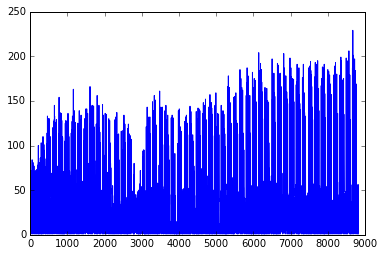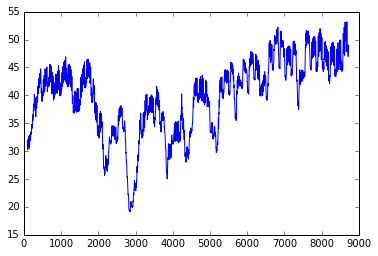In :
import time
import datetime
from collections import defaultdict
import matplotlib.pyplot as plt
import numpy
import urllib
import scipy.optimize

In :
events = {}

In :
# Data is from:
# https://s3.amazonaws.com/babs-open-data/babs_open_data_year_1.zip

In :
# Extract the time information from the events
for f in [
#    "201508_trip_data.csv",
#    "201608_trip_data.csv",
"201408_babs_open_data/201408_trip_data.csv",
"201402_babs_open_data/201402_trip_data.csv"
]:
f = open(f, 'r')
for l in f:
l = l.split(',')
tripID = l
timeString = l
timeUnix = time.mktime(datetime.datetime.strptime(timeString, "%m/%d/%Y %H:%M").timetuple())
events[tripID] = [timeUnix, timeString]

In :
# Find the earliest event
earliest = None
for event in events:
if earliest == None or events[event] < earliest:
earliest = events[event]

In :
earliestTime = earliest

In :
hourly = defaultdict(int)

In :
# Count events by hour
for event in events:
t = events[event]
hour = int(t - earliestTime) // (60*60)
hourly[hour] += 1

In :
f = open("hourly.json", 'w')
f.write(str(dict(hourly)) + '\n')

Out:
76832
In :
hourly = eval(open("hourly.json").read())

In :
# Observations sorted by hour
hourlySorted = []
for h in hourly:
hourlySorted.append((h,hourly[h]))

In :
hourlySorted.sort()

In :
X = [x for x in hourlySorted]
Y = [x for x in hourlySorted]

In :
# Plot the raw observation data
plt.plot(X,Y)
plt.show()In :
# Plot using a sliding window
sliding = []

In :
wSize = 24*7

In :
tSum = sum([x for x in hourlySorted[:wSize]])
rSum = sum([x for x in hourlySorted[:wSize]])

In :
for i in range(wSize,len(hourlySorted)-1):
tSum += hourlySorted[i] - hourlySorted[i-wSize]
rSum += hourlySorted[i] - hourlySorted[i-wSize]
sliding.append((tSum*1.0/wSize,rSum*1.0/wSize))

In :
X = [x for x in sliding]
Y = [x for x in sliding]

In :
plt.plot(X,Y)
plt.show()In :
# Autoregressive features
def feature(hour):
previousHours = []
for i in [1,2,3,4,5,24,24*7,24*7*365]:
previousHour = hour - i
previousHourExists = previousHour in hourly
if previousHourExists:
# Use the feature if it doesn't exist
previousHours += [0, hourly[previousHour]]
else:
# Otherwise add a "missing value" indicator
previousHours += [1, 0]
return previousHours

In :
X = [feature(x) for x in hourly]
y = [hourly[x] for x in hourly]

In :
theta,residuals,rank,s = numpy.linalg.lstsq(X, y)

/usr/local/lib/python3.5/dist-packages/ipykernel_launcher.py:1: FutureWarning: rcond parameter will change to the default of machine precision times max(M, N) where M and N are the input matrix dimensions.
To use the future default and silence this warning we advise to pass rcond=None, to keep using the old, explicitly pass rcond=-1.
"""Entry point for launching an IPython kernel.

In :
theta

Out:
array([-1.01926529,  0.47807283, -3.09032484, -0.28047782, -0.37190452,
0.10121378,  4.34069395,  0.02242255,  3.72092429, -0.02606211,
-0.17356089,  0.15790027,  6.0151401 ,  0.52526083,  0.56372825,
0.        ])